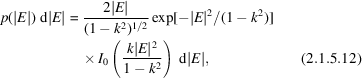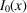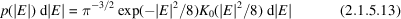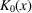International
Tables for
Crystallography
Volume B
Reciprocal space
Edited by U. Shmueli

International Tables for Crystallography (2006). Vol. B. ch. 2.1, p. 196   | 1 | 2 |

## Section 2.1.5.4. Other ideal distributions

U. Shmuelia* and A. J. C. Wilsonb

aSchool of Chemistry, Tel Aviv University, Tel Aviv 69 978, Israel, and bSt John's College, Cambridge, England
Correspondence e-mail:  ushmueli@post.tau.ac.il

#### 2.1.5.4. Other ideal distributions

| top | pdf |

The distributions just derived are asymptotic, as they are limiting values for large N. They are the only ideal distributions, in this sense, when there is only strict crystallographic symmetry and no dispersion. However, other ideal (asymptotic) distributions arise when there is noncrystallographic symmetry, or if there is dispersion. The subcentric distribution,whereis a modified Bessel function of the first kind and k is the ratio of the scattering from the centrosymmetric part to the total scattering, arises when a noncentrosymmetric crystal contains centrosymmetric parts or when dispersion introduces effective noncentrosymmetry into the scattering from a centrosymmetric crystal (Srinivasan & Parthasarathy, 1976, ch. III; Wilson, 1980a,b; Shmueli & Wilson, 1983). The bicentric distributionarises, for example, when the asymmetric unit in a centrosymmetric crystal is a centrosymmetric molecule' (Lipson & Woolfson, 1952);is a modified Bessel function of the second kind. There are higher hypercentric, hyperparallel and sesquicentric analogues (Wilson, 1952; Rogers & Wilson, 1953; Wilson, 1956). The ideal subcentric and bicentric distributions are expressed in terms of known functions, but the higher hypercentric and the sesquicentric distributions have so far been studied only through their moments and integral representations. Certain hypersymmetric distributions can be expressed in terms of Meijer's G functions (Wilson, 1987b).

### ReferencesLipson, H. & Woolfson, M. M. (1952). An extension of the use of intensity statistics. Acta Cryst. 5, 680–682.Google ScholarRogers, D. & Wilson, A. J. C. (1953). The probability distribution of X-ray intensities. V. A note on some hypersymmetric distributions. Acta Cryst. 6, 439–449.Google ScholarShmueli, U. & Wilson, A. J. C. (1983). Generalized intensity statistics: the subcentric distribution and effects of dispersion. Acta Cryst. A39, 225–233.Google ScholarSrinivasan, R. & Parthasarathy, S. (1976). Some statistical applications in X-ray crystallography. Oxford: Pergamon Press. Google ScholarWilson, A. J. C. (1952). Hypercentric and hyperparallel distributions of X-ray intensities. Research (London), 5, 588–589.Google ScholarWilson, A. J. C. (1956). The probability distribution of X-ray intensities. VII. Some sesquicentric distributions. Acta Cryst. 9, 143–144.Google ScholarWilson, A. J. C. (1980a). Relationship between observed' and `true' intensity: effects of various counting modes. Acta Cryst. A36, 929–936.Google ScholarWilson, A. J. C. (1980b). Effect of dispersion on the probability distribution of X-ray reflections. Acta Cryst. A36, 945–946.Google ScholarWilson, A. J. C. (1987b). Functional form of the ideal hypersymmetric distributions of structure factors. Acta Cryst. A43, 554–556.Google Scholar